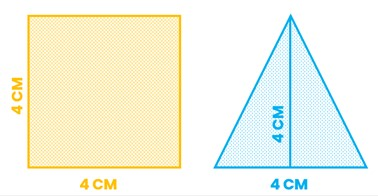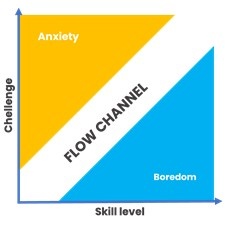# Why Do I Need Cuemath Program?

350 views

## Why Cuemath

To decide whether you need a specialist math program for your child, here are some important questions that you need to ask yourself:

• What is considered as good math education? Is 100% score an indicator of holistic math learning?
• If your child is already interested in math and currently tops the class, how can you retain that enthusiasm?
• What would a math program for a child already scoring 100% look like?

### The traditional definition of math education and scoring 100%

Usually, the school system and the traditional notion of mathematics are limited to math concepts that are prescribed for the grade the student is in. In a lot of cases, math is taught as a set of rules and formulae to solve given problems using the given set of rules and formulae.

In such a system, students can score 100% if they:

• memorize the definitions and formulae that are given to them in the class.
• practice and remember step by step method of solving the problem to get to the answer. Any missing step can lead to a loss of score. So, students will have to learn through rote, to replicate the solution in exams.
• stick to the questions and solutions provided in the textbook and repeat the process multiple times. There is no incentive for going outside the textbooks to apply the concept.
• practice the important topics for the exam using the regular test papers. They can leave a couple of chapters that they have difficulty learning and still score 100%

In other words, students can score a 100% if they stick to what is being asked of them and replication solutions, step by step. This takes away the ability to adopt the right strategy for the right context. Lacking the understanding of algorithms and why something is true could be the reason for this.

Try this example:

Ask your child to solve this problem and show you the solution. Time them while they are working on it.

What is the total area of the square and the triangle put together?Step 1: Calculate the area of the square:
For the given square side (S) = 4 cms
Area of a square = S²
∴ Area of the given square = 4 x 4 = 16 sq. cm

Step 2: Calculate the area of the triangle
The base of the given triangle (b) = 4 cms
Height of the given triangle (h) = 4 cms
Area of a triangle = ½ x b x h
∴ Area of the given triangle = ½ x 4 x 4 = 8 sq. cm

Step 3: Add the two areas
Area of the given square = 16 sq. cm
Area of the given triangle = 8 sq. cm
Total area = 16 + 8 = 24 sq. cm

This method is needed and is the right way to solve it in the context of a school exam. But if the student is in a fast-paced environment like competitive exams or Olympiads, this method will slow them down. However, students will default to this method due to the force of habit.

### How to solve this faster?

The area of a square is S² and the area of a triangle is half the area of the square/rectangle that it can fit into.
So, the combined area of the square and the triangle is one and a half times the area of the square.
∴ the combined area is 1.5 (4 x 4) = 24 sq. cms

This is possible if the student understood why the area of a triangle is ½ x b x h & what could be the relationship between the area of a square and the area of a triangle with the same base. But in a traditional school system, the students would not have the time to explore and make these connections. Tuitions simply replicate what the schools do.

Someone who has a natural inclination to the subject will need to be challenged for better results:
Now, let’s assume that your child is already thinking outside the textbooks and explores the ‘why’ behind every formula, recognizes the patterns and loves to solve math problems. This enthusiasm needs to be retained by challenging them with higher complexity problems.

What you see below is a simplified model of ‘Flow’ from “Flow: The Psychology of Optimal Experience” written by a Hungarian Psychologist named Mihaly Csikszentmihalyi.Flow is a state where concentration and complete absorption into the activity at hand. It occurs when the level of challenge is matched with the level of skill level. In your child’s case, if he/she is not challenged enough, they may fall into the boredom spectrum and eventually lose interest in the subject.

So, like every star athlete, even students need a coach to challenge them by:

• Asking them to try as many methods as possible to solve a problem.
• Give stretch targets and encourage them to try harder.
• Praise them only when they have completed a new and more difficult task and recognize the effort

Unfortunately, these things seldom happen in traditional math learning programs.

A math program for achievers should be more challenging and holistic. Math is not just the theorems, formulae and rules. While they are very important for math learning, students should be encouraged to:

1. Understand the why, before they learn a theorem, formula or a rule and question why it is true in all cases before accepting it as a fact. They should learn through reasoning.
2. Try multiple methods to solve the same problem to understand how does a concept work. They should learn by doing.
3. Apply the knowledge to solve problems outside textbooks and think creatively to solve the problems where a concept is not already taught
4. Be quicker and faster in solving the problems by skipping several steps to arrive at the solution, when time is of the essence
5. Try and test multiple strategies before adopting a method to solve a problem and apply the right approach at the right time.

### Cuemath as a program is built on these very principles.

• It covers multiple domains such as Concepts (necessary for school and formal learning), Aptitude (for speed and accuracy) and Creative Reasoning (for thinking out of the box and solving difficult problems).
• Cuemath method insists upon asking ‘Why’ before accepting the ‘what’ and stresses upon doing various activities on your own to learn algorithms.
• Our teachers are trained to take up the role of a coach and challenge the children to the right amount to maintain their Flow.

#### So, in summary:

• You must think about the traditional notion of math learning and ensure your child is going beyond it to help learn math.
• They should be challenged enough to maintain their enthusiasm and interest in the subject.
• The math curriculum must include multiple math domains like concepts, aptitude and creative reasoning for a holistic math learning and Cuemath offers them all.
• The learning method should encourage all the above aspects and Cuemath method encompasses all the mentioned aspects

Related Articles
GIVE YOUR CHILD THE CUEMATH EDGE
Access Personalised Math learning through interactive worksheets, gamified concepts and grade-wise courses